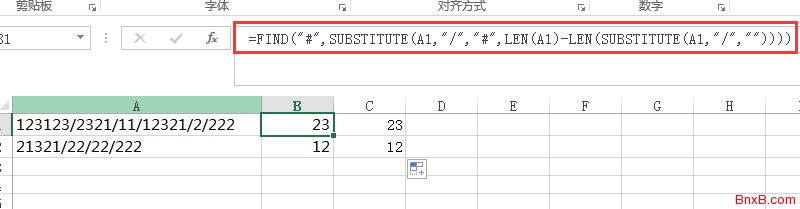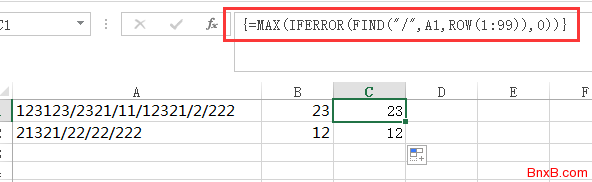• 导航
• .

# EXCEL中查找某个单元格内某个字符最后出现位置的公式

=FIND("#",SUBSTITUTE(A1,"要查找的那个字符","#",LEN(A1)-LEN(SUBSTITUTE(A1,"要查找的那个字符",""))))=MAX(IFERROR(FIND("要查找的那个字符",A1,ROW(1:99)),0))• ^